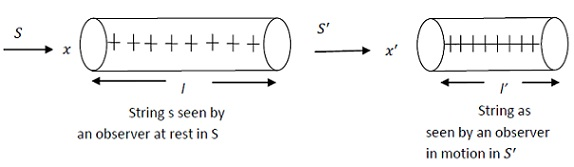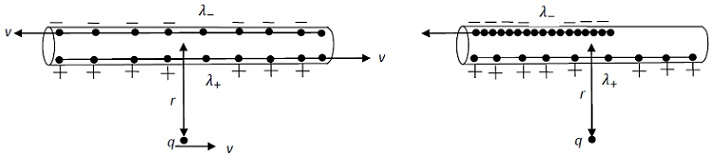#### Magnetism as a Relativistic Phenomenon, Physics tutorial

Electric Charges and Charge Density As Seen In Different Frames of Reference:

Electric Charges As Seen By Different Observers In Different Frames of Reference:

The total charge of isolated system is constant. This is statement of conservation of charge. It demands that quantity of charge which flows in isolated body should be exactly equal to amount which flows out of it.

For a neutral atom, number of protons in nucleus equals number of electrons in electron cloud. Now, in the atom, these protons and electrons are in motion, yet their charges are accurately balanced out. So, atom severely maintains electrical neutrality. Basic charge of the proton and electron is electronic charge; ±e. Net charge on the macroscopic object is integral multiple of electronic charge. Therefore, different observers in different frames of reference will count same number of electronic charges in any macroscopic object. In other words, if N electronic charges, Ne, comprise net charge Q on object as seen by the observer in S' frame, observer in frame will also see N electronic charges and net charge Q' of magnitude Ne. Thus, net charge of any macroscopic body is invariant under Lorentz transformation. Magnitude of electric charge is same irrespective of how fast it is moving.

Linear Charge Density As Seen By Different Observers In Different Frames of Reference:

Charge density depends on dimensions of object on which there is the charge distribution. For instance, linear charge distribution is charge per unit length. And lengths of objects appear shortened relative to the observer in frame of reference in which it is in motion (length contraction). So, linear charge density differs with variation of length of charge distribution.Consider the string of equally spaced charges at rest in S frame as shown in figure.

We will suppose that charges are close enough to form the continuous string of charge of length l. If charge of system is Q = ne, where N = 1,2,3..., then, linear charge density λ is given as:

λ = Q/l

in coulomb/metre(C/m) or Qλl in coulomb(c). If l = 1m, then λ = Ne C/m

Now, as seen in S' frame which is in motion to right along +x-axis at constant velocity v relative to S length l of charge string is shortened by factor β = √1 - v2/c2. That is l' = l√1-v2/c2, where l' is contracted length as seen in S' frame. Linear charge density λ' in S' frame is thus

λ' = Q/l' = Q/l(√(1 - v2/c2))

Therefore for unit length, i.e. l = 1m we have

λ' = Ne/√(1 - v2/c2) = γNe

Therefore, N charges appear squashed into the contracted length 1/γ = β = √1 - v2/c2

Thus conclude that linear charge density is not Lorentz invariant. Of course, by extension, surface and volume charge density is also not Lorentz invariant.

Electric and Magnetic Forces:

Parallel conductors carrying current in same direction apply attractive magnetic force on each other. Now, in current-carrying conductor, electrons are in motion relative to apparently stationary positive ionic cores or protons. Conventionally, direction of electric current in conductor is taken as direction of motion of the positive cores. Now show how magnetism arises, given electrostatics and relativity.Consider, once again, the string of equally spaced positive charges, this time moving steadily to right with the constant drift speed v. We, again suppose that charges are close enough to form continuous string of positive charges of linear charge density λ+. Of course, you also have string of negative charges with linear charge density λ-, moving in opposite direction with similar speed v. As string has no net charge, it is electrically neutral. Net current to right is I = 2λv

Now, consider the charge q at distance r perpendicular to conductor and moving to right with velocity, that we will assume to be v0. You know that electron drift speeds are very small compared with relativistic speed of q. There is no electrical force on q, because string, we have already agreed, is neutral as seen in S frame.

In S' frame, q is at rest but S' is moving at the speed v0 relative to S. Velocity of positive charges as observe in S' frame is

v+ = (v - v0/(1 - vv0/c2))

And velocity of negative charges as observe in S' frame is

v- = (v + v0/(1 + vv0/c2))

As you can see, v- is greater than v+, squashing up of negative charges is more severe than positive charges in S' frame. Wire will then carry the net negative charge. Therefore, we have

λ+ = +γ+λ0

And λ- = -γ-λ0 Here

γ+ = 1/√(1 - v2+/c2) and γ- = 1/√(1 - v2-/c2)

Now λ0 is charge density of positive line in its rest frame, so

λ = γλ0

γ = 1/√(1 - v2/c2)

Therefore we write

γ+ = γ(1 - vv0/c2)/√(1 - v02/c2)

γ- = γ(1 + vv0/c2)/√(1 - v02/c2)

Thus, net line charge as seen in S' is

λtot = λ+ + λ- = λ0+ - γ-) = -2λv0/c2(1 - v02/c2)

The consequence of unequal Lorentz contraction of positive and negative lines, otherwise neutral current-carrying wire in one frame of reference becomes charged in another reference frame.

To compute electric field due to this resultant charge, as seen in S' frame, we have

E = λtot/2πε0r

Electrical force due to resultant charge is

F = qE = (λv/πε0c2r)(qv0/√1 - v02/c2)

Now, from Newton's third law of motion, forces exist in pairs. Therefore, if there is force of electrical origin in S' frame, there should also force in S frame. Compute this force by applying transformation rules, that is,

F = γF' = √1 - (v02/c2)F' = (λv/πε0c2)(qv0/r)

Noting that λv = I, current and c2 = (ε0μ0)-1in equation we write

F = -(μ0I/2πr)qu = Bqv

Where B = (μ0I/2πr) is magnetic field as you can recognize. So, force as seen in S frame is the magnetic force. Therefore, conclude that given electrostatics and relativity, we get magnetism.

Tutorsglobe: A way to secure high grade in your curriculum (Online Tutoring)

Expand your confidence, grow study skills and improve your grades.

Since 2009, Tutorsglobe has proactively helped millions of students to get better grades in school, college or university and score well in competitive tests with live, one-on-one online tutoring.

Using an advanced developed tutoring system providing little or no wait time, the students are connected on-demand with a tutor at www.tutorsglobe.com. Students work one-on-one, in real-time with a tutor, communicating and studying using a virtual whiteboard technology.  Scientific and mathematical notation, symbols, geometric figures, graphing and freehand drawing can be rendered quickly and easily in the advanced whiteboard.

Free to know our price and packages for online physics tutoring. Chat with us or submit request at info@tutorsglobe.com